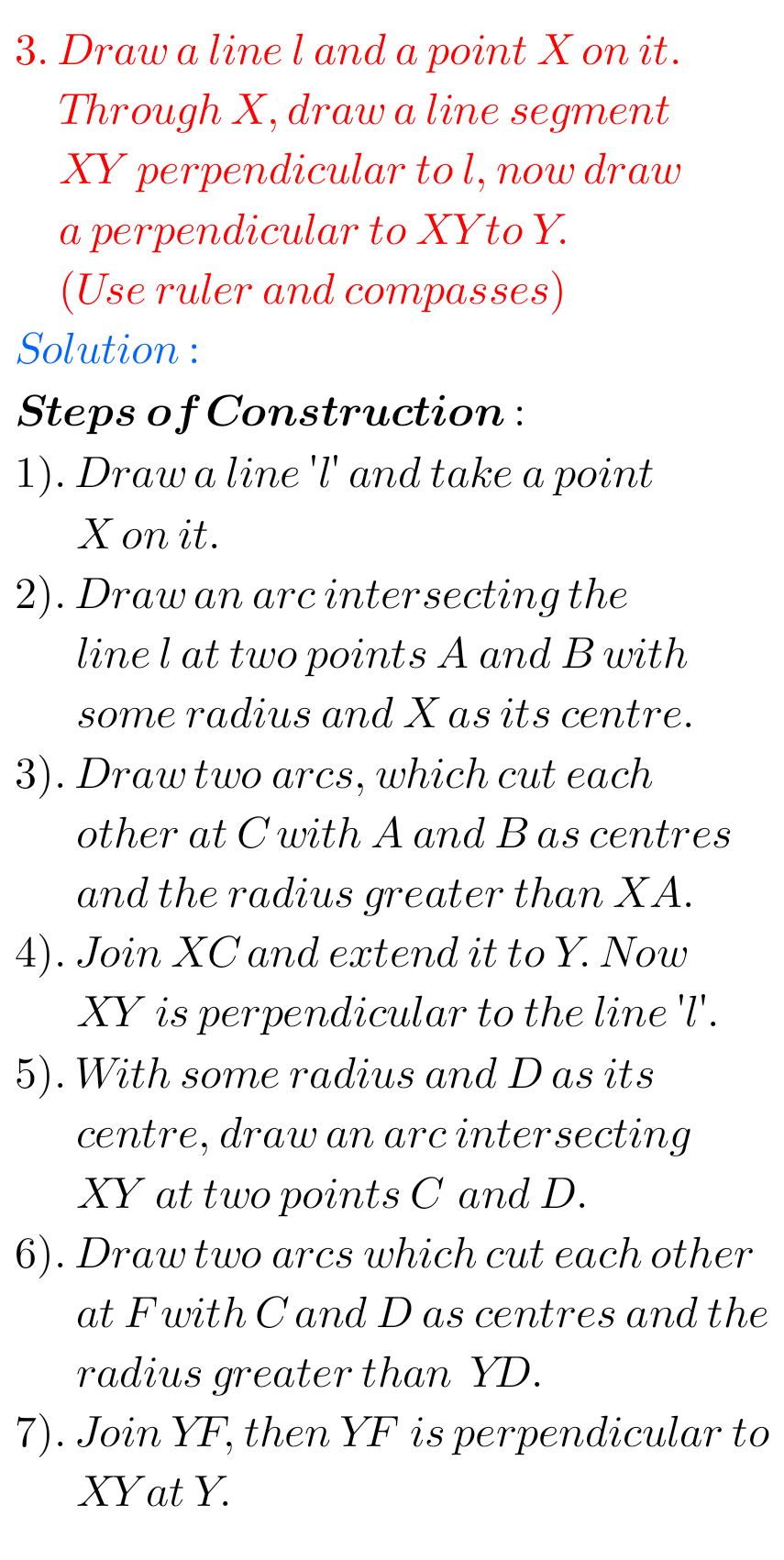## Ncert Maths Chapter 14 Practical Geometry Exercise 14.4 Solutions Class 6

Practical Geometry Solutions Exercise 14.4 Class 6 Ncert Ncert solutions for Mathematics Class 6th textbook Chapter 14 Practical Geometry Exercise 14.4 are given. First you study the textbook lesson Practical Geometry very well. You should also observe the example problem and solutions given in the textbook. Observe the given below solutions and try them in …